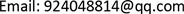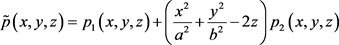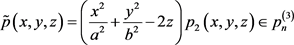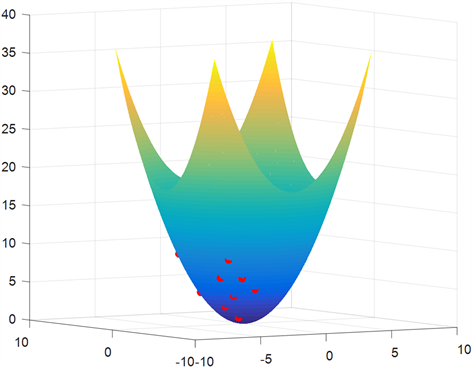﻿ 定义于椭圆抛物面上的多元Lagrange插值问题 Multivariate Lagrange Interpolation Defined on Elliptic Paraboloid

Vol. 08  No. 05 ( 2019 ), Article ID: 30282 , 5 pages
10.12677/AAM.2019.85105

Multivariate Lagrange Interpolation Defined on Elliptic Paraboloid

Jinshuang He, Lihong Cui

Liaoning Normal University, Dalian LiaoningReceived: Apr. 28th, 2019; accepted: May 13th, 2019; published: May 20th, 2019ABSTRACT

The multivariate Lagrange interpolation problem, which is usually defined on the elliptic paraboloid, is often studied in practical scientific research and production. Multivariate Lagrange interpolation is proposed to define the definition of Elliptic paraboloid, given to determine whether the node group on an elliptic paraboloid form judgment theorem and superposition method to construct interpolation regular set of nodes, finally is to implement the method.

Keywords:Elliptic Paraboloid, Multivariate Lagrange Interpolation, Regular Set of Nodes, Superposition Interpolation Method1. 引言

2. 基本定义和基本定理

$m=\left(\begin{array}{c}n+3\\ 3\end{array}\right)$，令 $Α={\left\{{Q}_{i}\right\}}_{i=1}^{m}$${R}^{3}$ 中m个互异点构成的点集，如果对于任意给定的数组 $\left\{{f}_{i}\in R|i=1,\cdots ,m\right\}$，恒存在唯一多项式 $p\left(x,y,z\right)\in {P}_{n}^{\left(3\right)}$，使之满足： $p\left({Q}_{i}\right)={f}_{i},i=1,\cdots ,m$，则称A为 ${P}_{n}^{\left(3\right)}$ 的一个唯一正解结点组    。

$p\left(x,y,z\right)={p}_{1}\left(x,y,z\right)+\left(\frac{{x}^{2}}{{a}^{2}}+\frac{{y}^{2}}{{b}^{2}}-2z\right){p}_{2}\left(x,y,z\right)$

$Α={\left\{{Q}_{i}\right\}}_{i=1}^{{\left(n+1\right)}^{2}}$ 为锥面F上的n次插值唯一正解结点组，平面 $p\left(x,y,z\right)\cap \text{A}=\varphi$ 与锥面F横截相交于

F上的 $m={\left(n+1\right)}^{2}$ 个互异点 ${\left\{{Q}_{i}\right\}}_{i=1}^{m}$ 能够做成定义于锥面F上的n次插值唯一正解结点组的充分必要条件是，若存在 $p\left(x,y,z\right)\in {p}_{n}^{\left(3\right)}$，满足 $p\left({Q}_{i}\right)=0,i=1,\cdots ,m$，蕴含如此的 $p\left(x,y,z\right)$ 在锥面F上恒为零。

${p}_{2}\left({Q}_{i}\right)=\frac{{f}_{i}-{p}_{1}\left({Q}_{i}\right)}{\frac{{x}_{i}^{2}}{{a}^{2}}+\frac{{y}_{i}^{2}}{{b}^{2}}-\frac{{z}_{i}^{2}}{{c}^{2}}}$

$i=m+1,\cdots ,r$，则多项式$p\left(x,y,z\right)=\stackrel{˜}{p}\left(x,y,z\right)=\left(\frac{{x}^{2}}{{a}^{2}}+\frac{{y}^{2}}{{b}^{2}}-2z\right){p}_{2}\left(x,y,z\right)\in {p}_{n}^{\left(3\right)}$

$p\left(x,y,z\right)$ 在锥面F上恒为零值。

$p\left(x,y,z\right)={a}_{1}{x}^{2}+{a}_{2}{y}^{2}+{a}_{3}{z}^{2}+{a}_{4}xy+{a}_{5}xz+{a}_{6}yz+{a}_{7}x+{a}_{8}y+{a}_{9}z+{a}_{10}$

$A=\left[\begin{array}{cccccccccc}9& 9& 81& 9& -27& -27& -3& -3& 9& 1\\ 4& 4& 16& -4& -8& 8& -2& 2& 4& 1\\ 4& 4& 16& 4& -8& -8& -2& -2& 4& 1\\ 1& 1& 1& 1& -1& -1& -1& -1& 1& 1\\ 9& 9& 81& -9& -27& 27& -3& 3& 9& 1\\ 4& 9& 42.25& 6& -13& -19.5& -2& -3& 6.5& 1\\ 9& 4& 42.25& 6& -19.5& -13& -3& -2& 6.5& 1\\ 1& 9& 25& 3& -5& -15& -1& -3& 5& 1\\ 9& 4& 42.25& 6& 19.5& 13& 3& 2& 6.5& 1\\ 4& 1& 6.25& 2& -5& -2.5& -2& -1& 2.5& 1\end{array}\right]$$X=\left[\begin{array}{c}{a}_{1}\\ {a}_{2}\\ {a}_{3}\\ a{}_{4}\\ {a}_{5}\\ {a}_{6}\\ {a}_{7}\\ {a}_{8}\\ {a}_{9}\\ {a}_{10}\end{array}\right]$$B=\left[\begin{array}{c}9.95\\ 4.90\\ 4.90\\ 1.73\\ 9.95\\ 7.43\\ 5.92\\ 7.43\\ 3.35\end{array}\right]$

$\begin{array}{c}f\left(x,y,z\right)=0.2322{x}^{2}+0.3054{y}^{2}+0.0261{z}^{2}+0.0033xy+1.1122xz\\ \text{\hspace{0.17em}}\text{\hspace{0.17em}}+0.1000yz+0.1160x+0.0778y+1.5252z+0.0252\end{array}$Figure 1. The effect picture of hyperboloid point taking

Multivariate Lagrange Interpolation Defined on Elliptic Paraboloid[J]. 应用数学进展, 2019, 08(05): 932-936. https://doi.org/10.12677/AAM.2019.85105

1. 1. 张步林. 关于二次锥面的讨论[J]. 成都纺织高等专科学校学报, 2007, 24(2).

2. 2. 赵兴仁. 利用锥面母线测量锥度的方法研究[J]. 新技术新工艺, 2017(9): 63-65.

3. 3. Higham, N.J. (1988) Fast Solution of Vandermonde-Like Systems Involving Orthogonal Polynomials. IMA Journal of Numerical Analysis, 8, 473-486.

4. 4. Bevilaqua, R., Bini, D., Capovani, M. and Menchi, O. (2003) Appunti di Calcolo Numerico. Chapter 5, p. 89.

5. 5. 戴聚岭. 多项式插值法在工程计算中应用[J]. 福建电脑, 2006(4): 178-179.

6. 6. 刘莹. 关于三元分次插值适定性的研究[J]. 应用数学进展, 2016, 5(4): 651-656.

7. 7. 邱爱保. 多元函数插值探讨[J]. 宜春学院学报(自然科学), 2007, 29(4): 32-33.